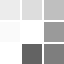## Survi Books International# Learn well series

Showing 1 to 12 of 27 (3 Pages)

₹126.00

₹140.00

Ex Tax: ₹126.00

..

₹84.00

₹140.00

Ex Tax: ₹84.00

..

₹126.00

₹140.00

Ex Tax: ₹126.00

..

₹84.00

₹140.00

Ex Tax: ₹84.00

..

₹126.00

₹140.00

Ex Tax: ₹126.00

..

₹84.00

₹140.00

Ex Tax: ₹84.00

..

#### Learn Well Swar Lehar

₹130.50

₹145.00

Ex Tax: ₹130.50

Swar Lehar is the Hindi language book for teaching Swar of Hindi language. ..

#### Learning Arithmetic 1

₹135.00

₹150.00

Ex Tax: ₹135.00

Learning Arithmetic Part 1 and 2  consists of basic mathematics concepts e.g. addition and subtraction, etc. ..

#### Learning Arithmetic 1 (E-Book)

₹90.00

₹150.00

Ex Tax: ₹90.00

Learning Arithmetic Part 1 and 2  consists of basic mathematics concepts e.g. addition and subtraction, etc. ..

#### Learning Arithmetic 2

₹135.00

₹150.00

Ex Tax: ₹135.00

Learning Arithmetic Part 1 and 2  consists of basic mathematics concepts e.g. addition and subtraction, etc. ..

#### Learning Arithmetic 2 (E-Book)

₹90.00

₹150.00

Ex Tax: ₹90.00

Learning Arithmetic Part 1 and 2  consists of basic mathematics concepts e.g. addition and subtraction, etc. ..

#### Learning Numbers 1 to 100

₹135.00

₹150.00

Ex Tax: ₹135.00

Learning Numbers 1‐100: teaching numbers 1‐100...

Showing 1 to 12 of 27 (3 Pages)